# 【密码学】公钥密码

(46) 2023-10-19 09:01:01

### 相关原理

（一）DH协议

算法描述：用户A和用户B通过公开信道建立共同的密钥。

1.公用参数生成算法

这一步骤将为用户A和B生成公用的参数，执行如下操作：

①选择大素数p。②令g为Zp*(2≤g≤p-2)的生成元。

2.协商密钥

①用户A随机选择数x(1≤x≤p-2)，计算g^x modp，并将之发送给B。②用户B随机选择数y(1≤y≤p-2)，计算g^y modp，并将之发送给A。③用户B收到g^x modp后，计算共享的密钥K=(g ^x)^y modp。④用户A收到g^y modp后，计算共享的密钥K=(g ^y)^x modp。

（二）RSA密码

RSA加密算法是一种非对称加密算法，所谓非对称，就是指该算法加密和解密使用不同的密钥，即使用加密密钥进行加密、解密密钥进行解密。在RAS算法中，加密密钥（即公开密钥）PK是公开信息，而解密密钥（即秘密密钥）SK是需要保密的。加密算法E和解密算法D也都是公开的。虽然解密密钥SK是由公开密钥PK决定的，由于无法计算出大数n的欧拉函数phi(N)，所以不能根据PK计算出SK。也就是说，对极大整数做因数分解的难度决定了RSA算法的可靠性。理论上，只要其钥匙的长度n足够长，用RSA加密的信息实际上是不能被解破的。

RSA算法通常是先生成一对RSA密钥，其中之一是保密密钥，由用户保存；另一个为公开密钥，可对外公开。为提高保密强度，RSA密钥至少为500位长，一般推荐使用1024位。这就使加密的计算量很大。为减少计算量，在传送信息时，常采用传统加密方法与公开密钥加密方法相结合的方式，即信息采用改进的DES或IDEA密钥加密，然后使用RSA密钥加密对话密钥和信息摘要。对方收到信息后，用不同的密钥解密并可核对信息摘要。

RSA的加密过程可以使用一个通式来表达：密文=明文^EmodN   也就是说RSA加密是对明文的E次方后除以N后求余数的过程。从通式可知，只要知道E和N任何人都可以进行RSA加密了，所以说E、N是RSA加密的密钥，也就是说E和N的组合就是公钥。其中E必须满足两个条件：E是一个比1大比L小的数，E和L的最大公约数为1（L 是 p－1 和 q－1的最小公倍数）。

RSA的解密过程可以使用一个通式来表达：明文=密文^DmodN   也就是说对密文进行D次方后除以N的余数就是明文，这就是RSA解密过程。知道D和N就能进行解密密文了，所以D和N的组合就是私钥。数D是由数E计算出来的，数D必须保证足够大。D、E和L之间必须满足：1 < D < L；E*D mod L ＝ 1。

（三）椭圆曲线密码

椭圆加密算法ECC，是基于椭圆曲线数学理论的一种非对称加密算法(公钥加密算法)，与RSA相比可以使用更短的密钥实现与RSA相当或者更高的安全性。这里的椭圆曲线是指具有以下形式的三次方程:y^2+axy+by=x^3+cx^2+dx+e其中a、b、c、d、e是满足某些简单条件的实数。定义中包括一个称之为无穷远点的元素，记为O，椭圆曲线上的加法运算定义如下：如果其上的3个点位于同一直线上，那么它们的和为O，进一步可以定义如下运算律：①O为加法单位元，即对椭圆曲线上的任意一点P，有P+O=P。②设P1=(x，y)是椭圆曲线上一点，它的加法逆元定义为P2=-P1=（x,-y）。③设Q和R是椭圆曲线上x坐标不同的两点，Q+R的定义如下：画一条通过Q、R的直线，与椭圆曲线交于P1（这一交点是唯一的，除非所做的直线是Q点或R点的切线，此时分别取P1=Q和P1=R）。由Q+R+P1=O，得Q+R=-P1。④点Q的倍数定义如下：在Q点做椭圆曲线的一条切线,设切线与椭圆曲线交于点S，定义2Q=Q＋Q=-S，类似的，可以定义3Q=Q+Q+Q，等等。以上定义的加法具有加法运算的一般性质，如交换律、结合律等。

椭圆曲线密码体制：设P∈E（Fp），点O是P的倍数，即存在正整数x，使Q=dP，则椭圆曲线离散对数问题（ECPLP）是指由给定的P和Q确定出x。系统构造：选取基域Fp，椭圆曲线E，在E上选择阶为素数n的点P（xp,yp）。公开信息为：基域Fp、椭圆曲线E、点P及其阶n。密钥生成：用户 Alice随机选取整数d，1<d≤n—1，计算Q=dP，将点Q作为公开密钥，整数d作为秘密密钥。加密与解密：若要给 Alice发送秘密信息M，需执行以下步骤：①将明文M表示为域Fp中的一个元素m。②在[1,n-1]内随机选择整数k。③计算点（x1,y1）=kP。④计算点（x2,y2）=kQ，若x2=0，则重新选择k。⑤计算c=mx2.⑥将（x1,y1,c）发送给 Alice。Alice收到密文后，利用秘密密钥d，计算：d（x1,y1）= dkP= K（dP）=kQ=（x2,y2） 。

（四）ELGamal加密体制

EIGamal加密算法是一种非对称加密算法，可以应用在任意一个循环群（Cyclic Group)上。在群中有的运算求解很困难，这些运算通常与求解离散对数(Discrete Iogarithm)相关，求解的困难程度决定了算法的安全性。其中涉及以下几个重要的数学定义。

公钥生成：①选取一个循环群G，且循环群G的阶数为q。②选择一个随机数x，l<x<q-1。③计算h=g ^xmodq。h和g，G，q就构成公钥；x是保密的，x与h，g，G，q一起构成密钥。

公钥加密：①选取一个随机数y，1<y<q-1。②计算c1=g^ymodq③计算s=h^y=（g^x）^y=g^（x*y）mod q。④加密数字m得c2=m *smodq。c1、c2构成加密结果，交给私钥解密

私钥解密：①通过c1计算得到s=c1^x=(g^y)^x=g（xy）mod q。②计算c2(s^-1)=(m*s)(s^-l)(md q)，得到明文数字m。

### 实现过程

**（一）DH协议   **

基于大整数库GMP，使用自带的函数实现随机数生成、大素数生成和求本原根，接着按照密钥协商顺序实现算法。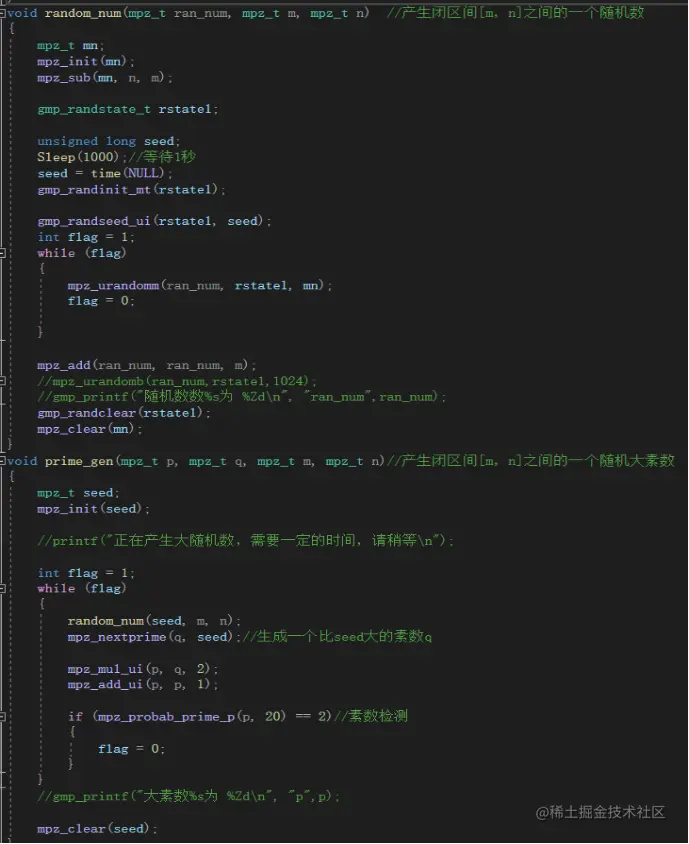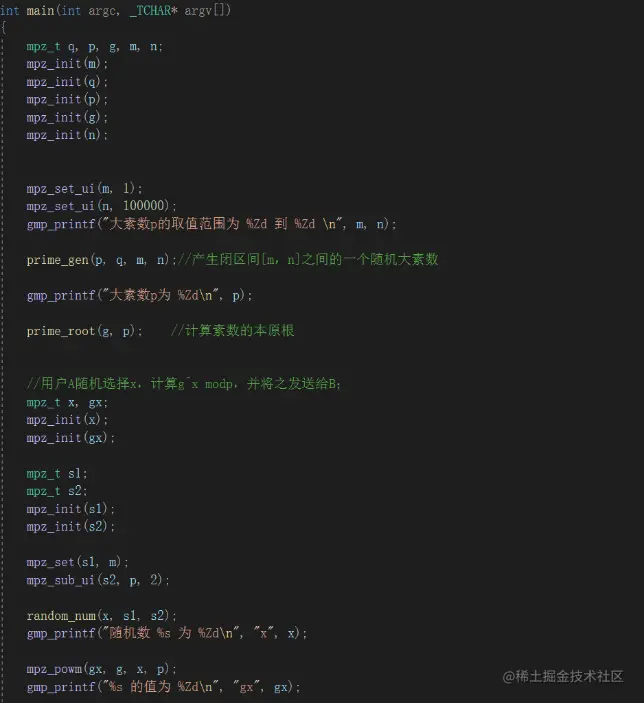（二）RSA密码

RSA加密算法的过程如下：

①取两个随机大素数p和q（保密）。

②计算公开的模数r=pq（公开）。

③计算秘密的欧拉函数φ(r)=(p-1)(q-1)（保密)，两个素数p和q不再需要，为了不泄露最好丢弃。④随机选取整数e，满足 gcd(e,φ (r))=1（公开e，加密密钥）。

⑤计算d，满足de=1(modφ(r))（保密d，解密密钥，陷门信息）。

⑥将明文（其值的范围在0到r-1之间）按模为r自乘e次幂以完成加密操作，从而产生密文y(其值也在0到r-1范围内)：y=x^e（mod r）

⑦将密文y按模为r自乘d次幂，完成解密操作：x=y^d （mod r）

``````int candp(int a, int n, int p){//数据处理函数，实现幂的取余运算

int r=1;

n=n+1;

while(n!=1){

r=(r*a)%p;

n--;     }

return r;

}

int fun(int x, int y){  //x与y的互素判断

int t;

while(y){

t=x;

x=y;

y=t%y;  }

if(x==1)

return 0; //x与y互素时返回0

else

return 1; //x与y不互素时返回1

}

void main(){

int p,q,e,d,m,n,z,c,r;

cout<<"①输入两个素数p,q: ";

cin>>p>>q;

n=p*q;

z=(p-1)*(q-1);

cout<<"②计算n=pq="<<n<<", z=(p-1)*(q-1)="<<z<<endl;

cout<<"③输入公钥e: ";

cin>>e;

if(e<1 || e>z || fun(e,z)){//选取e: e<n,e与z互素

printf("e不合要求，重新输人:");

scanf("%d", &e);

}

d=1;

while(((e*d)%z)!=1)//计算私钥d

d++;

cout<<"④满足ed modz=1的d: "<<d<<endl;

cout<<"⑤公钥("<<n<<","<<e<<"),私钥("<<n<<","<<d<<")"<<endl;

cout<<"1.加密"<<endl<<"2.解密"<<endl<<"3.退出"<<endl;

while(1){

printf("选择你执行的操作:");

cin>>r;

switch(r){

case 1:

printf("请输入明文m: ");

scanf("%d", &m);

c=candp(m, e, n);

printf("密文为%d\n",c);  //c=m^e modn

break;

case 2:

printf("请输入密文c: ");

scanf("%d", &c);

m=candp(c, d, n);

printf("明文为%d\n", m); //m=c^d modn

break;

case 3:

return;

default:

printf("输入错误,请重新输入:\n");

}  }  }
``````

（三）椭圆曲线密码

①用户A选定一条适合加密的椭圆曲线Ep(a,b)(如：y2=x3+ax+b)，并取椭圆曲线上一点，作为基点G。

②用户A选择一个私有密钥k，并生成公开密钥K=kG。

③用户A将Ep(a,b)和点K，G传给用户B。

④用户B接到信息后 ，将待传输的明文编码到Ep(a,b)上一点M，并产生一个随机整数r（r<n）。

⑤用户B计算点C1=M+rK；C2=rG。

⑥用户B将C1、C2传给用户A。

⑦用户A接到信息后，计算C1-kC2，结果就是点M。因为C1-kC2=M+rK-k(rG)=M+rK-r(kG) =M。再对点M进行解码就可以得到明文。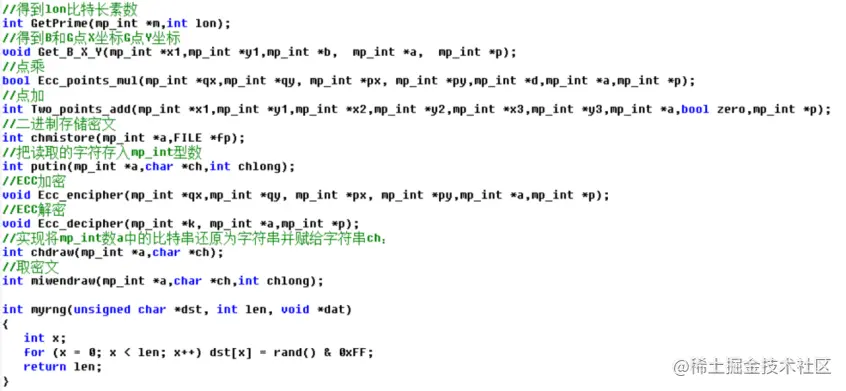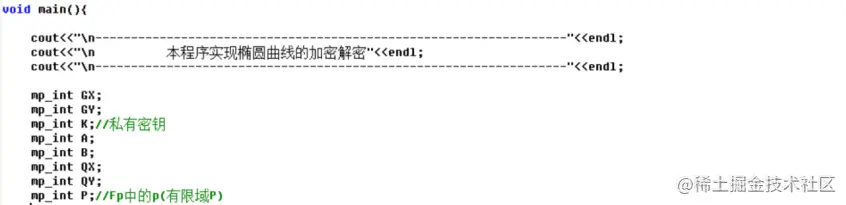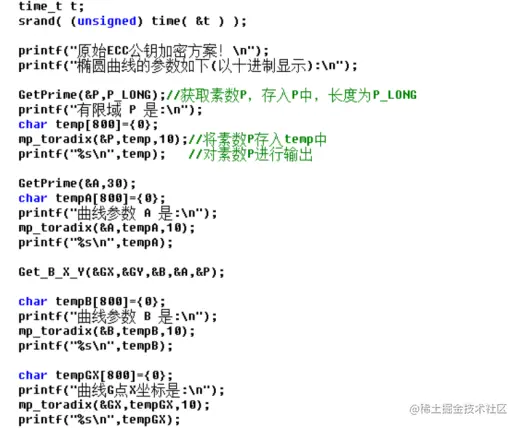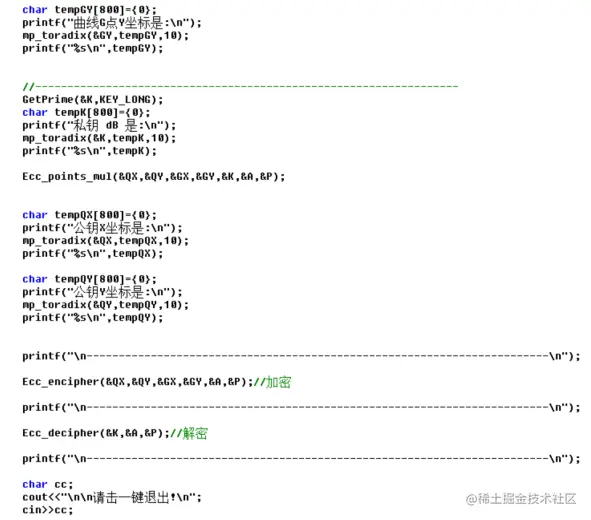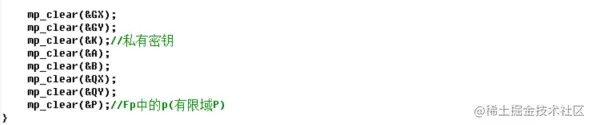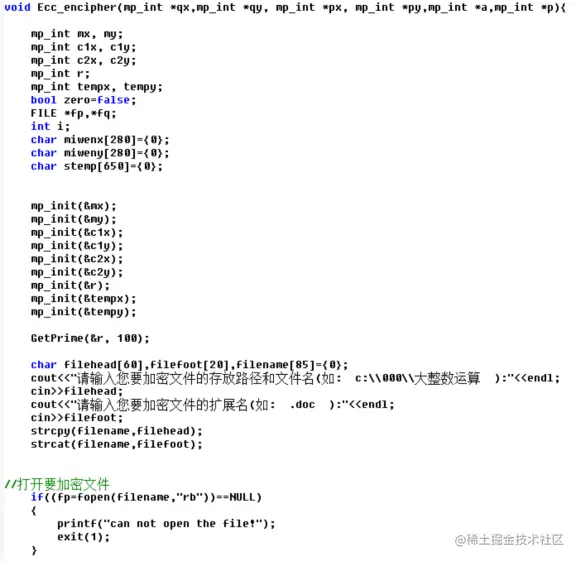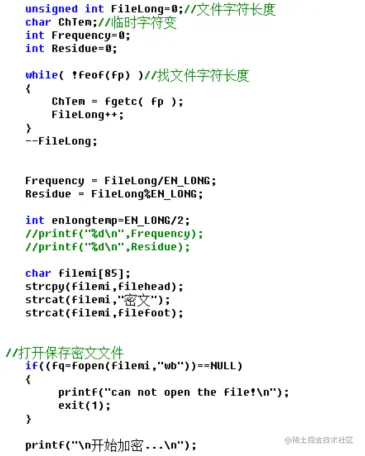（四）ELGamal加密体制

①密钥产生过程：选择素数p、g、随机整数x、秘密随机整数y，计算h = g^x mod p。h作为公开密钥，x作为秘密密钥。

②加密过程：明文消息m，随机选一整数y<p-1，计算c1 = g^y mod p，c2 = (m* h^ymodp)modp，密文为c=(c1,c2)。

③解密过程：m=(c2/c1^x)modp =mmodp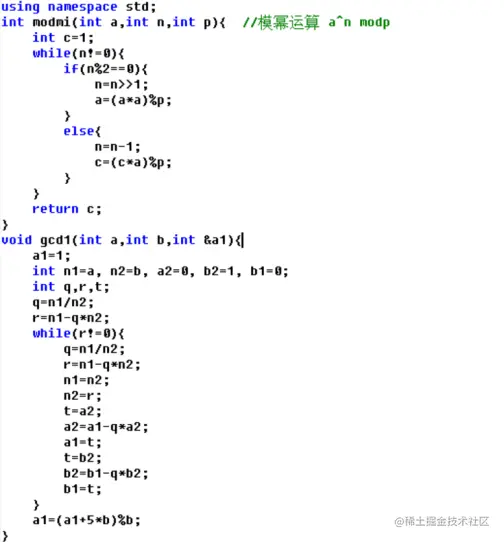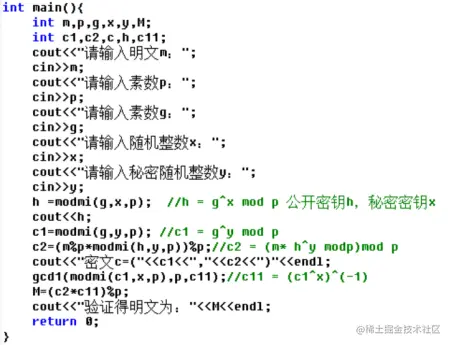### 结果测试

（一）DH协议

运行程序，结果如下图1所示。选取p=69263，计算其本原根g=19360。随机选择数x=11913，那么g ^x mod p=35861；随机选择数y=49075，那么g ^y mod p=21039。用户B计算的共享密钥Kb=54047，同样用户A计算的共享密钥Ka=54047。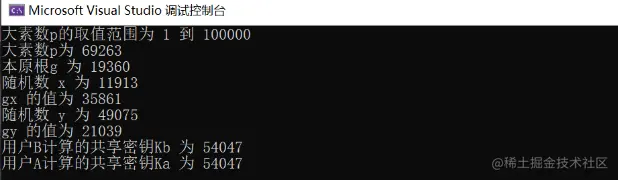（二）RSA密码

算法测试结果如下图2所示。首先输入两个素数p和q分别为17和11，得到n=1711=187,φ（n）=1610=160。输入e为7，7d=160*k+1得到d=23。此时公钥为（187,7），私钥为（187,23）。选择加密，明文m为71，得到密文c=m^e modn=71^7mod187=113；选择解密，密文113，得到明文m=c^d modn=113^23mod187=71。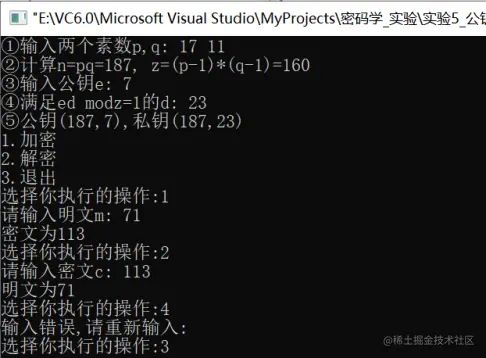（三）椭圆曲线密码

运行程序如下图3，输入要加密的文件路径“E:\测试\1.txt”,加密完成后，加密文件存放在1密文.txt中；输入要解密的文件路径“E:\测试\1密文.txt”，解密完成后，解密文件存放在1密文解密.txt中，结果如下图4所示。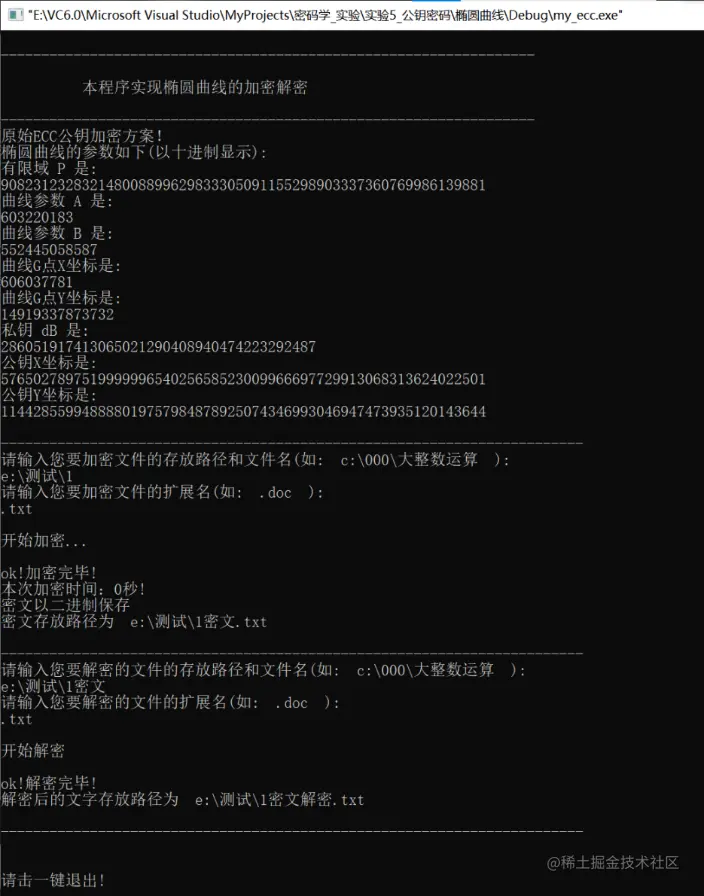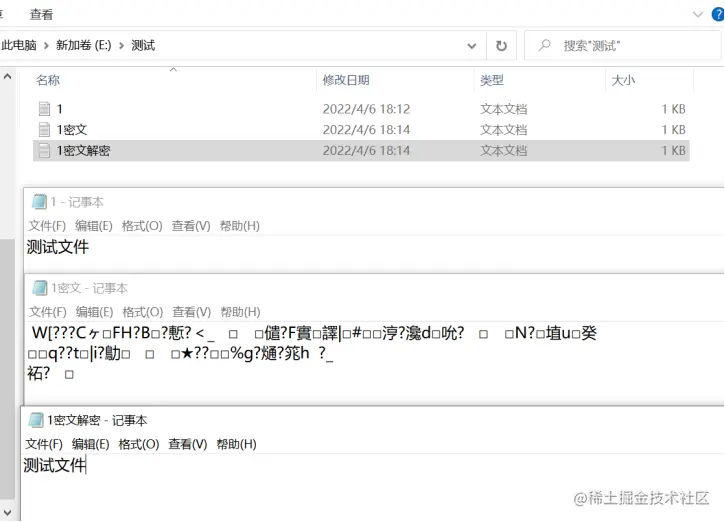（四）ELGamal加密体制

运行程序后如下图5所示，输入明文m、素数p、g、随机整数x、秘密随机整数y，得到h = g^x mod p=449^12mod509=438，c1 = g^y mod p=449^18mod509=231，c2 = (m* h^y modp)mod p=(100438^18 mod509)mod509=492，c=(c1，c2)=(231，492)，验证得明文m=(c2(c1^x)^(-1))%p=100。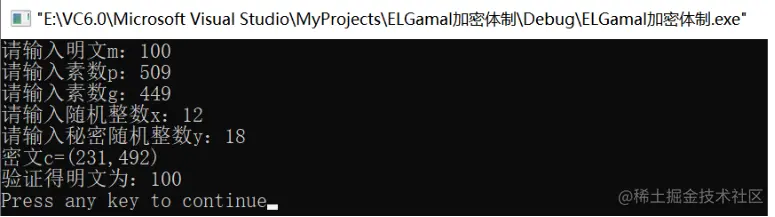THE END